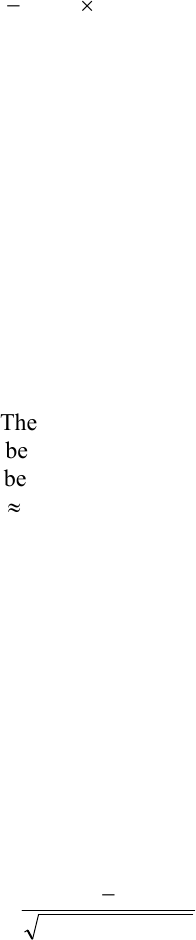Class Notes (1,100,000)
CA (650,000)
UTSC (30,000)
ATA (10)
Lecture 2

# MGEB12H3 Lecture Notes - Lecture 2: Type I And Type Ii Errors, Null Hypothesis, VarianceExam

Department
Economics for Management Studies
Course Code
MGEB12H3
Professor
ATA
Lecture
2

This preview shows half of the first page. to view the full 3 pages of the document.Page 1 of 3
TUTORIAL-2
1. A random sample of 20 observations taken from a normally distributed population
revealed a sample mean of 65 and a sample variance of 16. The lower limit of a 90%
confidence interval for the population mean would equal: (B)
A. 63.247
B. 63.454
C. 63.528
D. 66.188
E. 68.129
)20(/4729.165 sqrt
Questions 2-3: A box of cereal is listed as containing 10 ounces of oat-nut clusters. A
consumer-rights group is worried that the cereal company is systematically under-filing
the boxes. They found that a random sample of 26 boxes had an average weight of 9.876
ounces with a sample standard deviation of s = 0.483.
2. The null and alternative hypotheses are: (A)
A. H0: μ ≥ 10; H1: μ < 10
B. H0: μ ≠ 10; H1: μ = 10
C. H0: μ = 10; H1: μ ≠ 10
D. H0: μ = 10; H1: μ ≥ 10
E. H0: μ = 10; H1: μ > 10
3. The p-value for the test is approximately: (D)
A. between .01 and .05
B. between .05 and .075
C. 095.0
D. >.1
E. >.2
4. For testing the hypotheses H0: P = .75 versus H1: P > .75 at 5% significance level, we
obtain a sample of n = 100 and p = .83. The Type-I error for this test is approximately:
(B)
A. 0.025
B. 0.032
C. 0.050
D. 0.068
E. 0.082
847.1
100/25.075.0
75.083.0
Z